### A brief history of pi: part 2

by @ulaulaman about #piday #pi #MachinFormula #EulerIdentity
Today is the pi day, so I continue the brief history of $\pi$
After the introduction of $\pi$ in mathematics, one of the quest linked with the calculation of its digits is the research about its nature, or in other words what kind of number it is. Numbers classification is simple for all: we start with natural numbers (positive and negative), and so we can define rational numbers, as the numbers generated by the ratio between two natural numbers. Every rational number could be expressed like $\frac{a}{b}$, with $a$, $b$ natural and $b$ not null.
Johann Heinrich Lambert was the first to show the irrational nature of $\pi$ in 1761 in Mémoire sur quelques propriétés remarquables des quantités transcendantes circulaires et logarithmiques: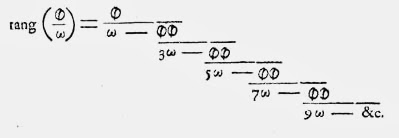commons
that could be written in this way: $\tan(x) = \cfrac{x}{1 - \cfrac{x^2}{3 - \cfrac{x^2}{5 - \cfrac{x^2}{7 - {}\ddots}}}}$ Lamberd proved that if $x$ is not null and rational, then the previous expression must be irrational. So the irrationality of $\pi$ follows from $\tan (\pi /4) = 1$. A good synthesis of Lambert's proof is on The world of $\pi$.
In 1997 Laczkovich proposed a simplification of this demonstration, while another variation was proposed in 2009 by Li Zhou, using the integral calculus. In particular the second demonstration is inspired by the proof that Charles Hermite written in two letters to Paul Gordan and Carl Borchardt in 1873. Following Harold Jeffreys in Scinetific interference (1973), a simplification of this proof, that used a reductio ab adsurdum is proposed by Mary Cartwright.
Another proof of one page about the irrationality of $\pi$ is dued by Ivan Niven in 1946.
At the other hand, the transcendence of $\pi$ is a direct consequence of the Lindemann-Weierstrass theorem:
If $\alpha_1$, $\cdots$, $\alpha_n$ are algebraic numbers that are linearly independent over rationals, then $e^{\alpha_1}$, $\cdots$, $e^{\alpha_n}$ are algebraically independent over rationals.
where an algebraic number is the solution of a polynomial equation with rational coefficients.
In 1882 Lindemann, using this theorem, showed that $e$ is transcendental, and, like a consequence of the Euler's identity, also $\pi$ is transcendental.
James Constant. Elementary proof that $\pi$ is irrational
Xavier Gourdon, Pascal Sebah. Numbers, constants and computation

Laczkovich M. (1997). On Lambert's Proof of the Irrationality of π, The American Mathematical Monthly, 104 (5) 439-443. DOI:
Li Zhou (2009). Irrationality proofs à la Hermite, arXiv:
Zhou L. & Markov L. (2010). Recurrent Proofs of the Irrationality of Certain Trigonometric Values, American Mathematical Monthly, 117 (4) 360-362. DOI: (arXiv)
Niven I. (1947). A simple proof that $\pi$ is irrational, Bulletin of the American Mathematical Society, 53 (6) 509-510. DOI:
Chow T.Y. (1999). What is a Closed-Form Number?, The American Mathematical Monthly, 106 (5) 440. DOI: (pdf)
The $\pi$ formulas that I examined last year are only a little subset of the complete set of pi formulas. This year I would examine some others, starting from the Machin formula: $\frac{1}{4} \pi = 4 \tan^{-1} \frac{1}{5} - \tan^{-1} \frac{1}{239}$ There are other three Machin formulas, that you can see them at MathWorld.
Another historical formula for $\pi$ is the Leibniz-Gregory formula: $\frac{\pi}{4} = \sum_{k=1}^n \frac{(-1)^{k+1}}{2k-1}$ This series is also known as the Leibniz series: only in a subsequent period it is associated to James Gregory's work, that rediscovered and published in 1668 a series that it was discovered by Madhava from Sangamagrama, an indian mathematician and astronomer of 14th century.
The formula could be modified in order to accelerate its convergence, using the $\zeta (s)$ of Riemann: $\pi = \sum_{k=1}^\infty \frac{3^k-1}{4^k} \zeta (k+1)$ Another historical series was discovered in 1717 by Abarham Sharp: $\pi = \sum_{k=0}^\infty \frac{2 (-1)^k 3^{1/2 - k}}{2k+1}$ Also Euler discovered a series about $\pi$: the great swiss mathematician not only named the number $\pi$, or found the identity that linked the most important math constants (and that in some sense summarize the math itself) $e^{i \pi} + 1 = 0$ but discovered also the following product of sequences, where $p_n$ is the $n_{th}$ prime number: $\pi \frac{2}{\prod_{n=1}^\infty \left ( 1 + \frac{\sin \left ( \frac{1}{2} \pi p_n \right )}{p_n} \right )}$ This formula could be also plotted: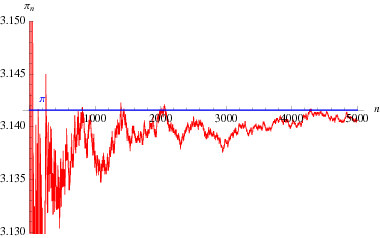Bailey D.H., Plouffe S.M., Borwein P.B. & Borwein J.M. (1997). The quest for PI, The Mathematical Intelligencer, 19 (1) 50-56. DOI: (pdf)
Jesus Guillera (2008). History of the formulas and algorithms for pi, La Gaceta de la RSME, 10 (2007) 159-178, arXiv:
Philippe Flajolet, Ilan Vard. Zeta Function Expansions of Classical Constants (pdf)
Wikipedia: Leibniz formula for $\pi$
Weisstein, Eric W. "Pi Formulas" From MathWorld--A Wolfram Web Resource.
One of the most interesting math challenge during the last century is the unsolved distribution of prime numbers. We have seen that the Riemann's zeta function, that plays a key role in the research, could also be used to calculate $\pi$.
So it is simple to imagine that also the distribution of digits of $\pi$ are not so random. Indeed Caldwell and Dudner play with the first 10 digits of $\pi$ in search of the prime numbers that we could construct with them. And they found 14 prime numbers!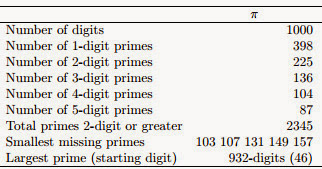And they also calculate the probability to find a pime number of some given digits in a given sequence of digits randomly extracted from $\pi$: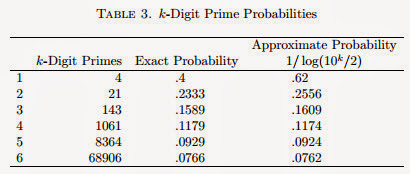This is an example of a classical problem without a clear practical utility, but that could allow to build new calculus tecnique, analitical or numerical, useful to solve multidisciplinary problems.
In every case, the digits of $\pi$ are infinity, and we know only about 5 trillions. Calculate them is really a great challenge, but also visualize them is not so simple, unless to use the technique based on the random walk.
This idea born around the quest of the normality of the transcendental numbers: a real number $a$ is called normal in base $b$ if every set of $m$ digits appears with a frequency equals to $1/b^m$. From this the step toward finding the distribution of digits of pi is short, and so the use of random walk in order to visualize $\pi$:Samuel Arbesman. A Random Walk with Pi
Walking on Real Numbers

Chris K. Caldwell, Harvey Dubner. Primes in Pi. Journal of Recreational Mathematics, Volume 29, Number 4, 1998 (pdf)
Aragón Artacho F.J., Bailey D.H., Borwein J.M. & Borwein P.B. (2013). Walking on Real Numbers, The Mathematical Intelligencer, 35 (1) 42-60. DOI:
Bailey D.H., Borwein J.M., Calude C.S., Dinneen M.J., Dumitrescu M. & Yee A. (2012). An Empirical Approach to the Normality of π, Experimental Mathematics, 21 (4) 375-384. DOI:
Aistleitner C. (2013). Normal Numbers and the Normality Measure, Combinatorics, Probability and Computing, 22 (03) 342-345. DOI: (arXiv)

#### 1 comment:

1.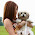well! π is most important part of the mathematics. Infect you can say that without “Pi” the math is incomplete subject. π is a mathematical constant, the ratio of a circle’s circumference to its diameter, approximately equal to 3.14159. The article which you have shared regarding “Pi” is very helpful for me. You have superb collection. I’m really glad to find this website. I must share this website with my friends. You have done good job. keep it up. You can see the helpful site regarding Math subject. Learn Math

Markup Key:
- <b>bold</b> = bold
- <i>italic</i> = italic
- <a href="http://www.fieldofscience.com/">FoS</a> = FoS